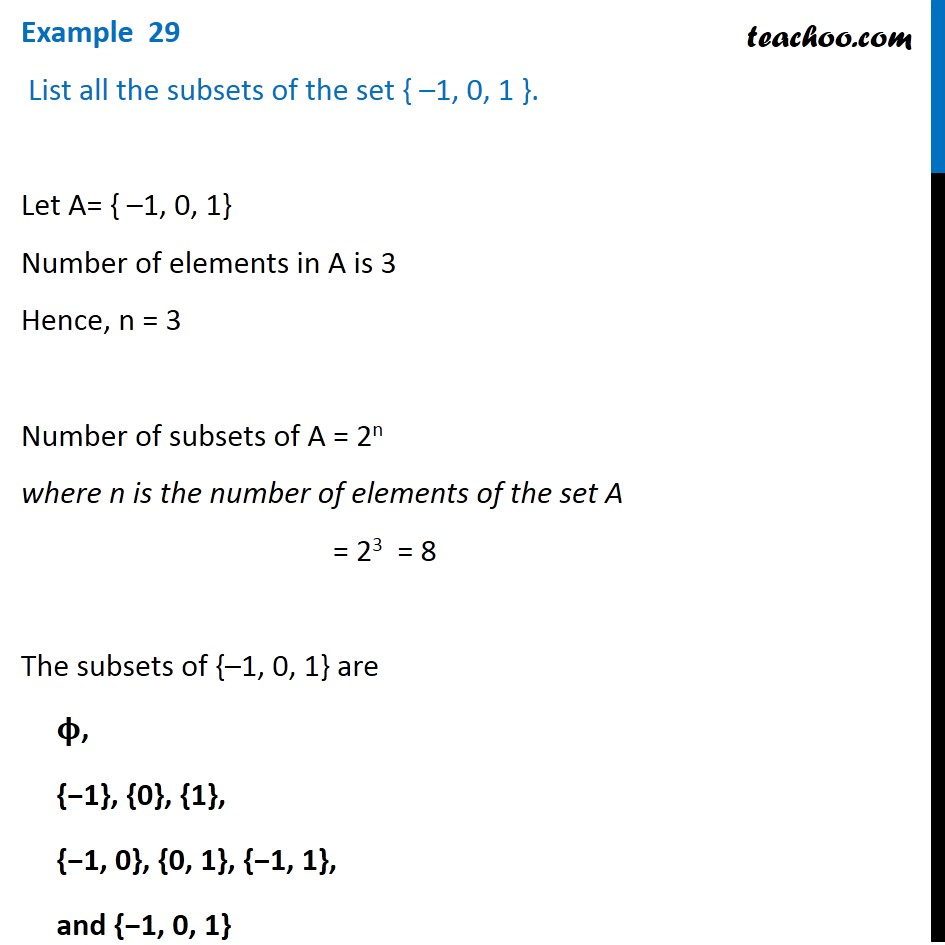1. Chapter 1 Class 11 Sets (Term 1)
2. Concept wise
3. Power Set

Transcript

Example 29 List all the subsets of the set { –1, 0, 1 }. Let A= { –1, 0, 1} Number of elements in A is 3 Hence, n = 3 Number of subsets of A = 2n where n is the number of elements of the set A = 23 = 8 The subsets of {–1, 0, 1} are 𝛟, {−1}, {0}, {1}, {−1, 0}, {0, 1}, {−1, 1}, and {−1, 0, 1}

Power Set